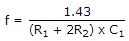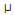# Electronics - Flip-Flops and Timers

### Exercise :: Flip-Flops and Timers - General Questions

6.

One example of the use of an S-R flip-flop is as a(n):

 A. transition pulse generator B. astable oscillator C. racer D. switch debouncer

Explanation:

No answer description available for this question. Let us discuss.

7.

If both inputs of an S-R NAND latch are LOW, what will happen to the output?

 A. The output would become unpredictable. B. The output will toggle. C. The output will reset. D. No change will occur in the output.

Explanation:

No answer description available for this question. Let us discuss.

8.

The equation for the output frequency of a 555 timer operating in the astable mode is:.
What value of C1 will be required if R1 = 1 k, R2 = 1 k, and f = 1 kHz?

 A. 0.33F B. 0.48F C. 480F D. 33 nF

Explanation:

No answer description available for this question. Let us discuss.

9.

An astable multivibrator is a circuit that:

 A. has two stable states B. is free-running C. produces a continuous output signal D. is free-running and produces a continuous output signal

Explanation:

No answer description available for this question. Let us discuss.

10.

What is another name for a one-shot?

 A. monostable B. bistable C. astable D. tristable